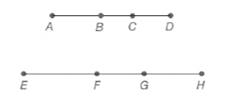Chapter 5.6, Problem 3EElementary Geometry For College St...

7th Edition
Alexander + 2 others
ISBN: 9781337614085

Solutions

Chapter
SectionElementary Geometry For College St...

7th Edition
Alexander + 2 others
ISBN: 9781337614085
Textbook Problem

Given that A B E F = B C F G = C D G H , are the following proportions true?a) A C E G = C D G H b) A B E F = B D F H

To determine

a)

To find:

Whether the proportion ACEG=CDGH is true or not.

Explanation

Given:

Given that ABEF=BCFG=CDGH for the line segments shown below.

Property used:

If ab=cd then a+cb+d=ab=cd.

Calculation:

Given: ABEF=BCFG=CDGH

Consider ABEF=B

To determine

b)

To find:

Whether the proportion ABEF=BDFH is true or not.

Still sussing out bartleby?

Check out a sample textbook solution.

See a sample solution

The Solution to Your Study Problems

Bartleby provides explanations to thousands of textbook problems written by our experts, many with advanced degrees!

Get Started

Simplify the expressions in Exercises 97106. 21/32122/321/3

Finite Mathematics and Applied Calculus (MindTap Course List)

Explain what is meant by the statement limx2f(x)=3.

Applied Calculus for the Managerial, Life, and Social Sciences: A Brief Approach

2. Define the terms population, sample, parameter and statistic.

Statistics for The Behavioral Sciences (MindTap Course List)

Evaluate the integrals in Problems 1-32. 14.

Mathematical Applications for the Management, Life, and Social Sciences

For f(x)=exx,f(x)= a) ex b) xex1x2 c) exxexx2 d) xexxx2

Study Guide for Stewart's Single Variable Calculus: Early Transcendentals, 8th

True or False:

Study Guide for Stewart's Multivariable Calculus, 8th